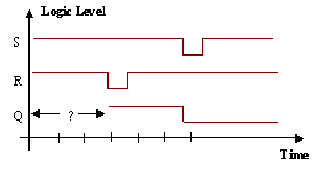## Featured post

### Top 5 books to refer for a VHDL beginner

VHDL (VHSIC-HDL, Very High-Speed Integrated Circuit Hardware Description Language) is a hardware description language used in electronic des...

## Tuesday, 27 September 2011

### R-S Flip FlopThe circuit of Fig.1 is called a SR flip-flop or bi-stable. We will consider its truth table, and immediately find that we have a problem. We can construct the table for the three states where at least one input is zero. This is because if any input to a nand gate is zero, the output will be one regardless of the other input, and we can therefore work around the loop to calculate all the values. However when R and S are 1 we cannot immediately calculate P and Q; so the only way we can analyze what happens is to look at the possible values that P and Q could hold. Thus we can expand the truth table to include a further two inputs Pp and Qp where the subscript p indicates the previous value.

 S R Pp Qp P Q 1 1 0 0 1 1 Unstable 1 1 0 1 0 1 Stable 1 1 1 0 1 0 Stable 1 1 1 1 0 0 Unstable

Here we see that there are two states where P=Pp and Q=Qp (1101 and 1110 respectively). These are therefore stable states. The other two states (1100 and 1111) are unstable, and using the simple model with time delay t will oscillate with a period of 2t. In practice, the circuit will fall into one of the two stable states rather than oscillate, since the time delays of the two nand gates will not be precisely the same. Which state if will finish in is non deterministic. In practice we are not interested in the non-deterministic states, only in the stable ones.This circuit can be considered to be a one bit memory circuit since Q can be set to one or zero. To see this we need to look at a sequence of inputs as shown in Fig.2. At the third time step we have the input 10 which puts the circuit into a known state and the output Q to 1. That value of Q is memorised and remains as long as the input is kept at 11. At the sixth time step the input 01 forces the output Q to be a 0, and as long as the input is held at 11 this 0 remains. This way of looking at the circuit gives rise to the names of the inputs S for Set and R for Reset, and so this flip flop is usually given the name R-S. The following three points should be noted. We are now describing the behaviour by means of a sequence of inputs, and for this reason, these circuits are referred to a sequential. Secondly, in all the cases of interest for this circuit P=Q'. Thirdly, an R-S flip flop can equivalently be built out of NOR gates.